# Long Strangle Payoff, Risk and Break-Even Points

This page explains long strangle profit and loss at expiration and the calculation of its risk and break-even points.

## Long Strangle Basic Characteristics

Strangle is a position made up of a long call option and a long put option with the same expiration date. It is similar to a straddle; the difference is that in a straddle both options have the same strike price, while in a strangle the call strike is higher than the put strike.

Long strangle (as well as long straddle) is a long volatility strategy. It is used when a trader expects the underlying to make a big move, but is unsure about the direction. It has limited risk and unlimited upside.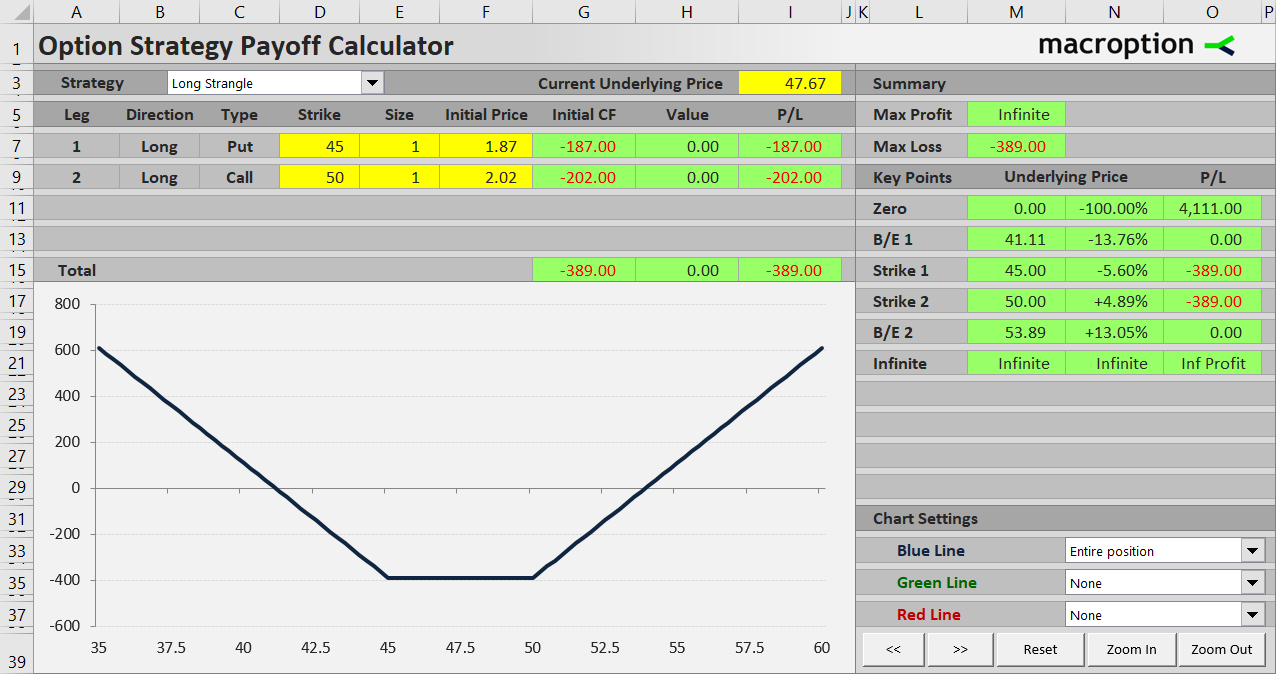If the underlying price moves far enough beyond one of the strikes (either up above the call strike or down below the put strike), one of the options gets in the money and, if its value exceeds the initial cost of both options, the trade makes a profit. On the contrary, if the volatility expectations don't materialize and underlying price stays between or near the strikes, it makes a loss.

We will use an example to explain the different scenarios in more detail; we will also calculate the risk (maximum loss) and break-even points.

## Long Strangle Example

Let's consider a long strangle position on a stock, currently trading at \$47.67, created by the following two transactions:

Buy a \$45 strike put option for \$1.87 per share, or \$187 for one contract.

Buy a \$50 strike call option on the same underlying, with the same expiration date, for \$2.02 per share, or \$202 for one contract.

Normally, the strikes are selected to have approximately the same distance from the underlying price at the time of opening the position. This ensures the trade has minimum directional bias.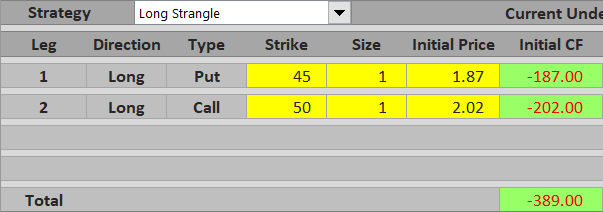## Initial Cost = Maximum Possible Loss

Because you are long both the options, the worst case scenario is that they both expire worthless. Therefore, the maximum you can lose from a long strangle trade is what you have paid for both options in the beginning. Risk is limited to initial cost of the position.

It is of course very easy to calculate:

Risk = initial cost = put initial cost + call initial cost

In our example:

Risk = initial cost = \$1.87 + \$2.02 = \$3.89 per share = \$389 for one contract

You will lose this amount if underlying price ends up between the strikes at expiration (or exactly at one of the strikes).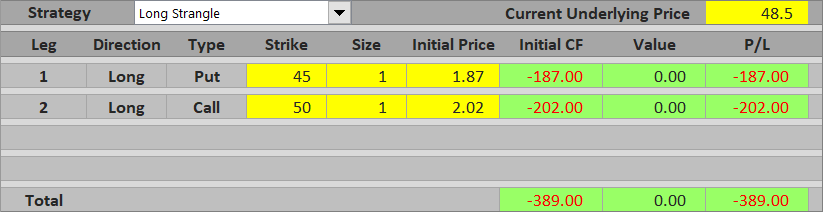## If Underlying Goes Up

If underlying price ends up above the call option strike at expiration, this option is in the money. You will exercise it and gain the difference between underlying price and the call strike.

For instance, it the stock in our example ends up at \$52.80, you will make \$2.80 per share or \$280 per contract. However, this is still less than what you have paid for the strangle (\$389) and therefore the overall result is a loss of \$389 – \$280 = \$109. The put option does not make any positive contribution, because it is out of the money, so the call option's value needs to pay for both options' initial cost.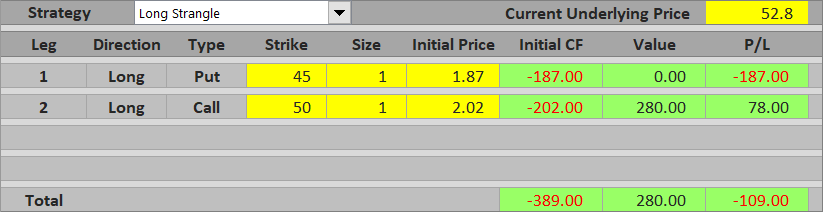To make a profit, the underlying would need to get even higher. If the stock ends up at \$55, the call would be worth \$500 and the trade's total P/L would be positive – a profit of \$500 – \$389 = \$111.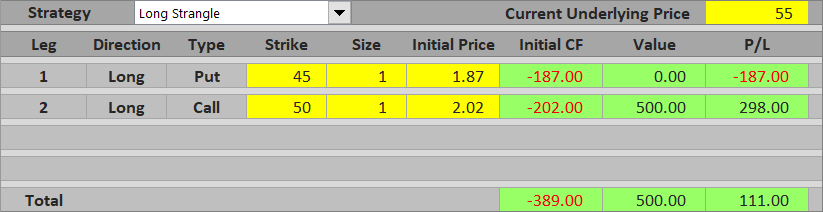## If Underlying Goes Down

It works in the same way if the underlying goes down, only the call and the put exchange their roles. If the stock falls below the put strike (\$45), the put is in the money and the call is worthless. For the strangle to make a profit overall, the put option's value must exceed the initial cost of both options.

For example, if the stock ends up at \$39 at expiration, the put is worth \$600, the call is worth zero, and therefore the trade's total profit equals \$600 – \$389 = \$211.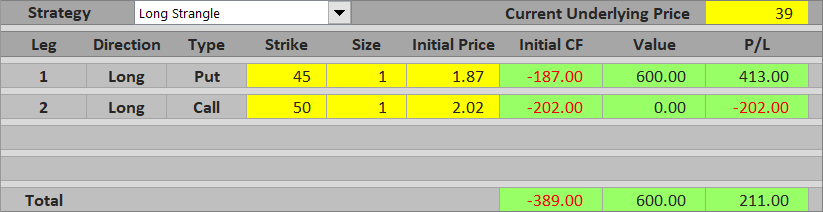## Long Strangle Break-Even Points

It is very useful to know the exact prices where the position starts to be profitable. This enables you to evaluate a potential trade before you enter it – to see how far the underlying price would have to move and whether expecting such a move is realistic.

These prices are known as break-even points and a long strangle has two of them – one down below the put strike and another one up above the call strike.

The lower break-even point is the exact underlying price where the put option's value at expiration equals the initial cost of both options.

B/E #1 = put strike – initial cost

Similarly, the upper break-even point is the price where the call option's value equals the initial cost of both options.

B/E #2 = call strike + initial cost

In our example:

B/E #1 = \$45 – \$3.89 = \$41.11

B/E #2 = \$50 + \$3.89 = \$53.89

You can see that the window of loss – the distance between the two break-evens – is much wider than the distance between strikes. In our example, where we entered the position when the stock was trading at \$47.67, its price has to go up by \$6.22 or down by \$6.56 for the strangle to make a profit – more than 13% up or down.

This is one disadvantage of a long strangle and the price you pay for the luxury of not having to predict the price direction. Only one of the two options can make money at a time, and it has to make enough to pay not only for itself, but also for the other option.

You can see this in the payoff diagram below, which also shows contribution of the individual legs – the green line is the long call, the red line is the long put, the thicker blue line is the entire strangle.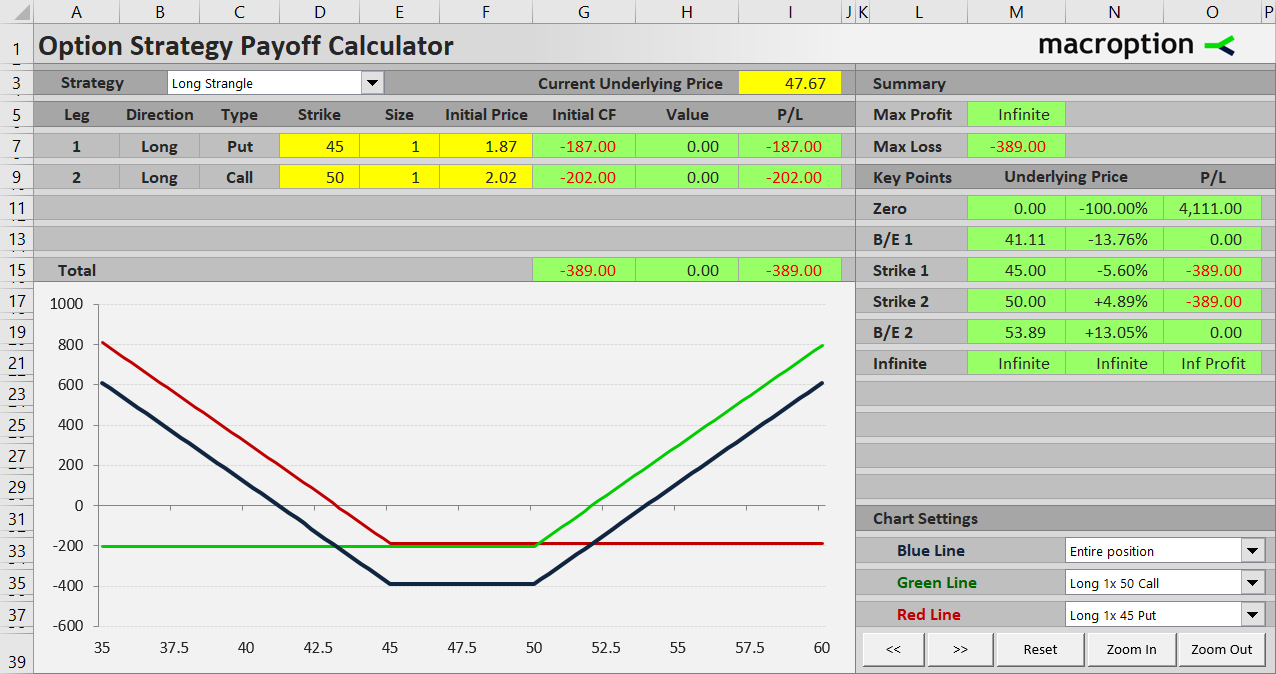In sum, long strangle should only be used when you believe that a very large move in either direction is likely.

## Similar Option Strategies

We have already mentioned that long strangle is very similar to long straddle. Both are non-directional long volatility strategies with limited risk and unlimited profit potential. As a result of the gap between strikes, long strangle has lower initial cost (and therefore risk), but its break-even points are further away, which means probability of profit is lower, other things being equal.

The inverse of long strangle is short strangle (short call + short put), which makes money when the underlying price stays between the two break-evens. It is a non-directional short volatility strategy with limited profit and unlimited risk. Similarly, the inverse of long straddle is short straddle, which has similar characteristics.

A little less popularly known strategy with payoff profile very similar to long strangle is long guts. It is also a non-directional long volatility strategy composed of a long call and long put with different strikes, but here the strikes are flipped – the long call strike is lower and the long put strike is higher. Its initial cost is higher (because both the options are typically in the money), but the overlap in their in the money price ranges compensates for that and net effect is very close to long strangle payoff.

By remaining on this website or using its content, you confirm that you have read and agree with the Terms of Use Agreement.

We are not liable for any damages resulting from using this website. Any information may be inaccurate or incomplete. See full Limitation of Liability.

Content may include affiliate links, which means we may earn commission if you buy on the linked website. See full Affiliate and Referral Disclosure.

We use cookies and similar technology to improve user experience and analyze traffic. See full Cookie Policy.